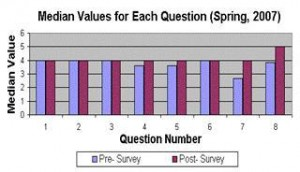## Measuring Student Perceptions of Confidence

November 14, 2008### From Pat Wagener, Can Problem Solving Become a Habit of Mind?

Pat says:

Students’ own perceptions of their math skills and abilities are often considered as anecdotal evidence, rather than factual information that has been quantified. In a meeting at the start of the Fall 2006 semester, this subject came up, and it occurred to me that this need not be the case. So I asked myself:

“Can students’ perception of their math skills and abilities be quantified, and measured with an acceptable level of statistical significance?”

And then,

“What is the research question that would enable me to answer this question?”

At first I thought to just have students assess their math skills in general. But then as I continued to think about this project, I realized it was important to know as much as possible about their self-assessment in connection with all the elements of the course: Multiple representation of mathematics, group work, solving word problems, computer aided instruction, and students making presentations of their solutions. And so I ended up with eight (8) areas for the students to assess.

A means to address this study was an attempt to quantify and measure each student’s self-assessed confidence learn in eight (8) specific areas or aspects of math and how they learn math. A survey would be given to the students early in the semester (pre-) and late in the semester (post-) to determine the change. These areas are as follows:

• math abilities and skills (in general)
• solving equations for a variable, like “x”
• using a graph to answer a question
• using a table to answer a question
• solving “word problems” (application problems)
• computer aided instruction (using a computer program for learning math)
• presenting solutions to the rest of the class
• group work as a means of helping to learn math
•With this method, I found that the anecdotal can become “factual” with a high degree confidence. Here are two key insights:

(1) Students’ self-perception changed very significantly in all questions (p-value<<0.01).

(2) An area of the greatest change (and most positive comments) was in the role of student presentations and self-confidence.